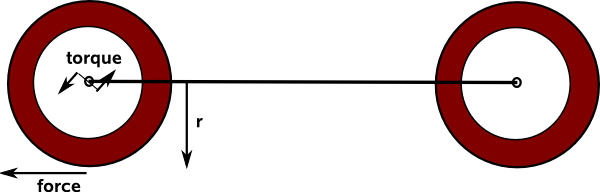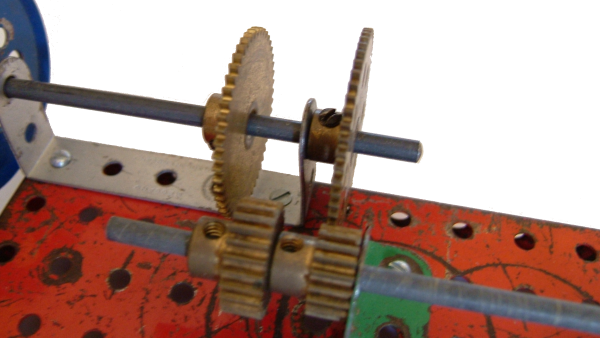# 3D Theory - Car Racing Game - Engine, Transmission and Traction

How much do we need to model the engine and transmission? We do to some extent because we need to know how fast the car can go and how quickly it can accelerate. The gear that is selected will affect the noise (loudness and pitch) of the engine. For a given engine there will be a graph of the torque that the engine can produce verses the speed of the engine. The gear is then selected to optimise the acceleration or speed depending on what is required.

This is quite a complicated subject because it would be nice to follow through cause and effect from say pressing foot on gas, through to engine speed, through to gear ratios and on to the resulting car speed. However things at the end of this chain, like the traction of the wheels, can effect the engine speed just as much from wheel spin to engine stall.

We therefore need to model the engine, transmission and traction as a single system combining together the equations for each sub system. We need to trace both the speed and force/torque through the whole system.

For example, think of the coupling between the axle parameters and the road speed we have:

torque/force:

torque = force × distance

F = T/r

speed:

linear velocity = angular velocity × distance

v = ω/rIf we trace through the torque and speed through all the systems we might start to build up a useful set of equations, but then we come across another problem:

The other thing that makes this difficult to model is that it can be very non-linear, for example, as we gradually increase the speed of the wheels then the road speed will increase in a linear way as described above. Then suddenly, at a point determined by the road surface (oil on road etc.), the wheels will start to spin, we are then in a completely different mode (wheel spin) given by a different set of equations. Even if we reduce the wheel speed below the point where they started spinning they will still spin (hysteresis effect).It therefore seems to me that we need to identify different modes for the system, such as,

1. engine stall
2. linear acceleration
3. wheel spin
4. breaking

and then derive a set of equations for each together with a set of criteria which switches between these modes.I'm not sure how accurately we need to model the various couplings that connect the engine to the wheels?